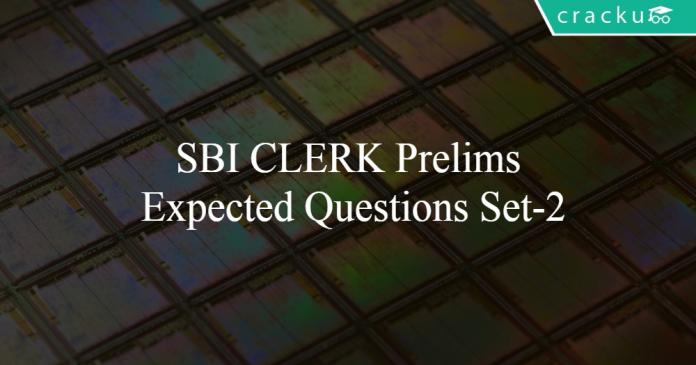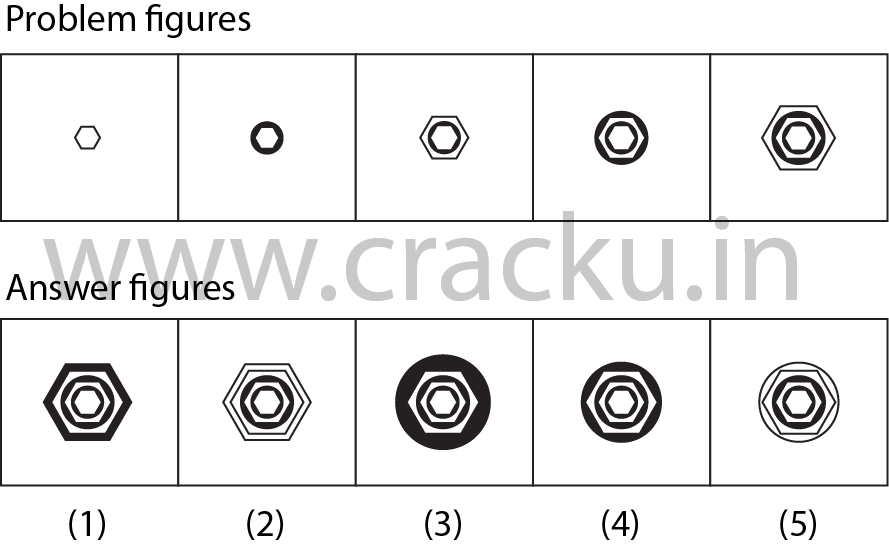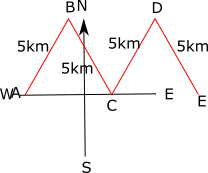# SBI CLERK Prelims Expected Questions 2019 Set-2

0
232## SBI CLERK Prelims Expected Questions 2019 Set-2

Download SBI Clerk Prelims Expected Questions Set-2 PDF for SBI Clerk Prelims exam. Very Important SBI Clerk Prelims  Expected Questions with solutions.

Question 1: Identify the part of the sentence that is grammatically wrong. If the sentence is grammatically correct, then select (No error) as the answer.After he is (1)/ finishes his work, (2)/ he plans to go (3)/ to Wonder La. (4)/ No Error (5).

a) 1

b) 2

c) 3

d) 4

e) 5

Question 2: In the question below, there is a word given in bold which is followed by five options. In each of the options, a pair of words is given which is either the pair of synonyms or antonyms or synonym & antonym of the word given in bold. Choose that pair as your answer.
Enervate

a) bolster : trigger

b) petrify : spook

c) soothe : fillip

d) prostrate : devitalize

e) stupefy : befuddle

Question 3: In the question below, there is a word given in bold which is followed by five options. In each of the options, a pair of words is given which is either the pair of synonyms or antonyms or synonym & antonym of the word given in bold. Choose that pair as your answer.
Dilettante

a) amateur : specialist

c) zestful : gloomy

d) slender : anorexic

e) sturdy : frail

Question 4: In the question below, there is a word given in bold which is followed by five options. In each of the options, a pair of words is given which is either the pair of synonyms or antonyms or synonym & antonym of the word given in bold. Choose that pair as your answer. Perspicacious

a) superlative : unexceptional

b) marvellous : inferior

c) heinous : egregious

d) astute : shrewd

e) pretentious : mediocre

Question 5: Four of the five are alike in a certain way and so form a group, identify the odd one out.

a) Pine

b) Bamboo

c) Deodar

d) Eucalyptus

e) Teak

Question 6: The ratio of ages of two friends A, B two years ago is 3:4. Sum of the present ages of A and C is 50 years and C is 11 years older than B. What is the present age of B?

a) 18 years

b) 21 years

c) 19 years

d) 22 years

e) 17 years

Question 7: $\large\sqrt{6084}\div\sqrt{2197}$ $+$ $\large\sqrt{54872}\times\sqrt{225}\div\sqrt{6859}$ $=$?

a) 34

b) 36

c) 28

d) 42

e) None of these

Question 8: The average marks of a class increased by $\large\frac{1}{3}$ when a student’s marks were wrongly entered as 64 instead of 46. The number of students in the class are

a) 54

b) 58

c) 63

d) 57

e) None of these

Question 9: Quantity 1:Last digit of $(313^{467})^{145}$
Quantity 2:Last digit of $(982^{347})^{254}$

a) Quantity 1 $\geq$ Quantity 2

b) Quantity 1 $\leq$ Quantity 2

c) Quantity 1 > Quantity 2

d) Quantity 1 < Quantity 2

e) Quantity 1 = Quantity 2

Question 10: P can finish the work in 16 days working on an average 5 hours/day. Q can finish the work in 24 days working 2.5 hours per day.If both P and Q together work for 12 hours a day, in how many days will the work be completed?

a) $\frac{20}{7}$ days

b) $\frac{10}{3}$ days

c) $\frac{22}{9}$ days

d) $\frac{43}{14}$ days

e) $\frac{47}{18}$ days

Question 11: If ‘+’ means father of, ‘-’ means the spouse of, ‘*’ means a sibling of and ‘/’ means daughter of, how is C related to A according to the following equation?
A – B + D * C + E

a) Son

b) Daughter

c) Son-in-law

d) Daughter-in-law

e) Cannot be determined

Question 12: Among five friends J, K, L, M and N each having a different number of pencils. K has more pencils than N but less than L. M has more pencils than L. J neither has the most nor the least number of pencils. Who amongst the following has the lowest number of pencils ?

a) Cannot be determined

b) M

c) N

d) L

e) K

Question 13: In a certain code language, ‘BRIGHT’ is coded as ‘CTLKMZ’. How will ‘PEOPLE’ be coded in the same language?

a) QRTKQS

b) QHSSRI

c) QGRTQK

d) RTSQPK

e) QSTPRQ

Question 14: A building B is present 5km North-East of a building A and Building C is present 5km South-East of B. Also building D is 5km to the North-East of C and 5km to the North-West of building E. What is the direction of building E in relation to A ?

a) East

b) South-East

c) North-West

d) West

e) cannot be determined

Question 15: Given below are five answer figures. Which of these five answer figures should come after the problem figures, if the sequence was continued?a) (1)

b) (2)

c) (3)

d) (4)

e) (5)

“After he finishes” is the correct grammatical construction.
=> Option A is the answer.

‘Enervate’ means to make someone feel drained of energy or vitality. ‘Prostrate’ and ‘devitalize’ are words with similar meanings.
Hence, option D is the correct answer.

‘Dilettante’ means a person who cultivates an area of interest, such as the arts, without real commitment or knowledge. ‘Amateur’ is the word with the same meaning and ‘specialist’ is the word with opposite the meaning.
Hence, option A is the correct answer.

‘Perspicacious’ means having a ready insight into and understanding of things. ‘Astute’ and ‘shrewd’ are words with similar meanings.
Hence, option D is the correct answer.

Bamboo is grass, others are trees.

Let us assume that a,b,c are the current ages of A,B,C.
a-2:b-2 = 3:4 =>4a = 3b+2…………..(I)
c = b+11
a+c = 50
a+b = 50-11 =39…………….(II)
From (I) and (II)
we get a = 17 and b = 22
Hence the current age of B is 22 years.

$\large\sqrt{6084}\div\sqrt{2197}$ $+$ $\large\sqrt{54872}\times\sqrt{225}\div\sqrt{6859} =$ $\Large\frac{78}{13}$ $+$ $\Large\frac{38\times15}{19}$

$=$ $\large6$ $+$ $\large30$ $=$ $\large36$

Let the number of students be ‘x’

Given that the average is increased by $\Large\frac{1}{3}$ due to the increase of $18$ marks ( $64$ $-$ $46$ $=$ $18$ marks )

Actual increase in marks $=$ $\Large\frac{1}{3}$ $\times$ x

$\Rightarrow$ $\Large\frac{1}{3}$ $\times$ x $=$ $18$

$\therefore$ Number of students(x) $=$ $54$

In quantity 1 it is the power cycle of 3 i.e last digits are 3,9,7,1,3,9,7,1…
Remainder when 467*145 is divided by 4 is same as remainder when 3*1 is divided by 4 since 3 and 1 are the remainders when 467 and 145 is divided by 4. Therefore 3 is the remainder and so last digit is 7.
In Quantity 2 it is the power cycle of 2 i.e last digits are 2,4,8,6,2,4,8,6…
Remainder when the number 347*254 is divided by 4 is 2 and so last digit will be $2^2 = 4$
Therefore Quantity 1 > Quantity 2

Work done per hour by P = $\frac{1}{16*5} = \frac{1}{80}$
Work done per hour by Q = $\frac{1}{24*2.5} = \frac{1}{60}$
Work done per hour if both work together = $\frac{1}{80} + \frac{1}{60} = \frac{140}{80*60}$
Number of hours taken to complete the work = $\frac{80*60}{140}$
Number of days taken to complete the work = $\frac{80*60}{140*12} = \frac{20}{7}$ days
Hence, option A is the right choice.

A – B means that A is the spouse of B.
B + D means that B is the father of D. Therefore, A must be the mother of D.
D * C means that D and C are siblings. Therefore, C must either be the son or the daughter of A.
C + E means that C is the father of E. Therefore, C is a male. C must be the son of A.
Therefore, option A is the right answer.

K has more pencils than N but less than L, => L > K > N.

M has more pencils than L, => M > L.

Combining above statements, we get : M > L > K > N

J neither has the most nor the least number of pencils.

Thus, N has the least number of pencils.

=> Ans – (C)

The pattern which is being followed here is that the first letter is being replaced by the letter which comes next in English alphabets. The second letter is replaced by the one which comes second after this letter in english alphabets. Similarly, the third is being replaced by the one which comes third and so on. For example
B + 1 = C
R + 2 = T
I + 3 = L
G + 4 = K
H + 5 = M
T + 6 = Z
Using the same logic, we can find the code word for PEOPLE to be ‘QGRTQK’.
Hence, option C is the correct answer.